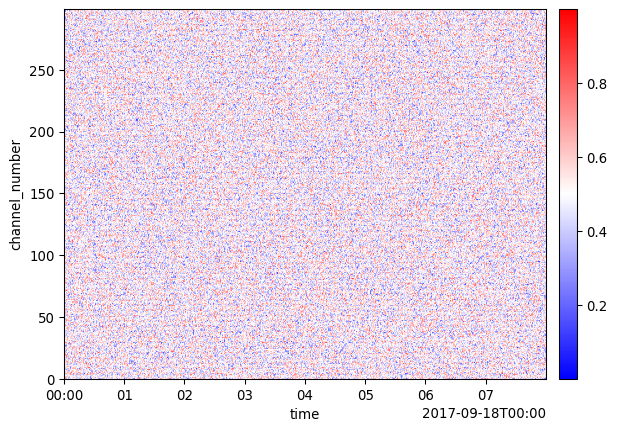# Plot Channel Number

Rather than plotting distance or depth, sometimes it is desirable to plot channel number (an index of the non-time dimension).

To do this involves three steps:

1. Adding a new coordinate called channel number
2. Setting the channel number as the dimension
3. Call plot function
``````import numpy as np

import dascore as dc

patch = dc.get_example_patch()

# get length of distance axis
dist_len = patch.coord_shapes["distance"]

# create range from 0 to dist_len
channel_number = np.arange(dist_len)

out = (
# first add channel number to coords.
patch.update_coords(channel_number=("distance", channel_number))
# then make channel number a dimensional coordinate
.set_dims(distance="channel_number")
)

# then plot
out.viz.waterfall();``````